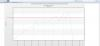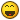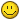## Recommended Posts

Hi, I have been able to create/render my chart fine. My only problem is that I can't figure out a way to add hover text to the points that aren't already labeled on the x axis. I was able to do this before with xml but I am trying the asp Class format now. Here is my code:

```<!DOCTYPE html PUBLIC "-//W3C//DTD XHTML 1.0 Transitional//EN" "http://www.w3.org/TR/xhtml1/DTD/xhtml1-transitional.dtd">
<%@ Language=VBScript %>

<html>

<meta http-equiv="Content-Type" content="text/html; charset=utf-8" />

<title></title>
<script type="text/javascript" src="jquery.min.js"></script>

<style type="text/css">

</style>
<script LANGUAGE="Javascript" SRC="FusionCharts.js"></SCRIPT>
<!-- #INCLUDE FILE="FusionCharts.asp" -->
<!-- #INCLUDE File="NewArray.asp" -->
<!-- #INCLUDE File="DBConn2.asp" -->
<!-- #INCLUDE File="RaceandColor.asp" -->
<!-- #INCLUDE File="FC_Colors.asp" -->
<!--#include file="FusionCharts_Gen.asp"-->

<body >

<div class="gen-chart-render"  >
<%
Dim strValue,strCategory, count, lc, hWeek,lWeek,month,year,cateCount,today, diffDate,sum,syear,ors2,monthsA(6), monthsB(3),x, isInA,isInB
dim FC
monthsA(0)=1
monthsA(1)=3
monthsA(2)=5
monthsA(3)=7
monthsA(4)=8
monthsA(5)=10
monthsA(6)=12
monthsB(0)=4
monthsB(1)=6
monthsB(2)=9
monthsB(3)=11

set FC = new FusionCharts
Call FC.setChartType("Line")
Call FC.setSize("90%","90%")
call FC.setSWFPath("../testfiles/")
Call FC.setID("NewChart")
lc=0
oConn.Open strConnQuery
today=Date()

year=2010
hWeek=31
lWeek=01
month=05
syear=2011
cateCount=0
count = 1
endDate=month&"/"&hWeek&"/"&year
do While DateDiff("d",CDate(endDate),today)>7
do While month<=12 And DateDiff("d",CDate(endDate),today)>40
diffDate = DateDiff("d",CDate(endDate),today)
x=0
isInA=false
isInB=false
do while x <= 6 and isInA = false

if monthsA(x) = month then
isInA = true
'response.Write(monthsA(x))
'response.Write("/br")
end if
x=x+1
loop
x=0
do while x <= 3 and isInB = false
if monthsB(x)=month then
'response.Write("arrayB")
isInB = true
' response.Write(monthsB(x))
end if
x=x+1
loop
'Starting new method for linegraph
sum = 0
if isInA then
hWeek=31
'response.Write("work")
strQuery = "select sum(zStratumID) as TotOutput from zRandomizationCode where zRandomizedOn BETWEEN '"&year&"-"&month&"-"&lweek&"' AND '"&year&"-"&month&"-"&hweek&"' "
Set oRs = oConn.Execute(strQuery)
sum = oRs("TotOutput")
If month =1 then
response.write("1st quarter")
Call FC.addChartData("" & sum & "","label = 1st Quarter of "&year&"","")
Elseif month =10 then
response.write("4th quarter")
Call FC.addChartData("" & sum & "","label = 4th Quarter of "&year&"","")
elseif month = 7 then
response.write("3rd quarter")
Call FC.addChartData("" & sum & "","label = 3rd Quarter of "&year&"","")
Else
End if
end if
If isInB then
' response.Write("nope")
hWeek=30
strQuery = "select sum(zStratumID) as TotOutput from zRandomizationCode where zRandomizedOn BETWEEN '"&year&"-"&month&"-"&lweek&"' AND '"&year&"-"&month&"-"&hweek&"' "
Set oRs = oConn.Execute(strQuery)
sum = oRs("TotOutput")
if month = 4 then
response.write("2nd quarter")
Call FC.addChartData("" & sum & "","label = 2nd Quarter of "&year&";hoverText=qwe;","")
else
Call FC.addChartData("" & sum & "","HoverText=qwe","")
end if
end if
if isInA = false and isInB=false then 'for leapyear can use % of year
hWeek=28
strQuery = "select sum(zStratumID) as TotOutput from zRandomizationCode where zRandomizedOn BETWEEN '"&year&"-"&month&"-"&lweek&"' AND '"&year&"-"&month&"-"&hweek&"' "
Set oRs = oConn.Execute(strQuery)
sum = oRs("TotOutput")
Call FC.addChartData("" & sum & "","","")
end if
month = month + 1
loop
month = 1
year=year+1
endDate=month&"/"&hWeek&"/"&year
Loop
strparam=" caption=Quarterly Enrollment; subcaption=Current; xAxisName=Months; yAxisName=# of People; hovercapbg=FFECAA; hovercapborder=F47E00; formatNumberScale='0'; decimalPrecision='0'; showvalues=0; numdivlines=5; numVdivlines=6; yaxisminvalue='0'; lineThickness=2; rotateNames='1'; baseFontSize='15'; animation=0; shadow =0; alpha=0; anchorAlpha='0';showshadow=0; anchorRadius = 2;hoverText=1;"
call FC.setChartParams(strparam)
call FC.SetRenderer( "javascript" )
call FC.renderChart(false)

Set oRs = Nothing
oConn.close
%>

</div>

</body>
</html>```

and here is a picture of my chart.##### Share on other sitesHi,

For custom tool-tip you would need to add toolText attribute in the set element.

You would need to add following line code into your project to have custom tool-tip on the data-plots.

Ref. code:

##### Share on other sites

Hi,

For custom tool-tip you would need to add toolText attribute in the set element.

You would need to add following line code into your project to have custom tool-tip on the data-plots.

Ref. code:

Great thanks so much!!!!Edited by Neverguesswho

##### Share on other sitesGreat thanks so much!!!!